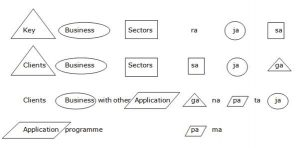## Coding and Decoding For SBI PO : Set – 37

1) How many such pairs of letters are there in the word EXAMINATION, each of which has as many letters between them in the word, as they have in the English alphabet?

a) None

b) One

c) Two

d) Three

e) More than three

c)

EXAINATION

E I and O N

2) If it is possible to make a three-digit number from the third, the fifth and the sixth digits of the number 30651972, using each digit only once which is the square of an odd number the last digit of that three-digit number is your answer. If no such number can be formed your answer is 4 and if more than one such number can be formed your answer is 8.

a) 1

b) 4

c) 8

d) 6

e) 9

c)

6, 1, 9

169 = (13)2

196 = (14)2

961 = (31)2

If more than one such number is formed. So the answer is 8.

3) If ROSE is coded as 6821, CHAIR is coded as 73456 and PREACH is coded as 961473, what will be the code for SEARCH?

a) 246173

b) 214673

c) 214763

d) 216473

e) None of these

b)

4) In a certain code language CAT is written as 3120; and DOG is written as 4157. Then which of the following is certainly the decoded form of 25144?

a) BEND

d) cant be determined

e) None of these

d)

Cannot be determined

5) By following certain logic MTUXTRVN is written as NUVXTQUM. How is ASUMNJKL written in that logic?

a) BTVMNIJK

b) BTVMNKLM

c) BTVNMIJK

d) ZRTMNIJK

e) None of these

a)

BTVMNIJK

D.6-10) Study the following information carefully and answer the given questions.

In a certain code language ‘key business sectors’ is written as ‘rajasa’, ‘clients business sectors’ is written as ‘sajaga’, ‘clients business with other application’ is written as ‘gana pa ta ja’ and ‘application programme’ is written as ‘pa ma’.

6) Which of the following is the code for ‘with application software’?

a) ta na sa

b) na paga

c) ta na ze

d) tapaca

e) Can’t be determined

e)7) What is the code for ‘other’?

a) na

b) ta

c) ma

d) Can’t be determined

e) None of these

d)8) Which of the following is the code for ‘with other key programme’?

a) na tamara

b) na tagapa

c) na tajasa

d) na masaga

e) None of these

a)9) What does ‘ra’ stand for?

a) sectors

b) key

d) clients

e) None of these

b)10) What is the code for ‘application’?

a) ra

b) ta

c) pa

d) ma

e) ga

c)